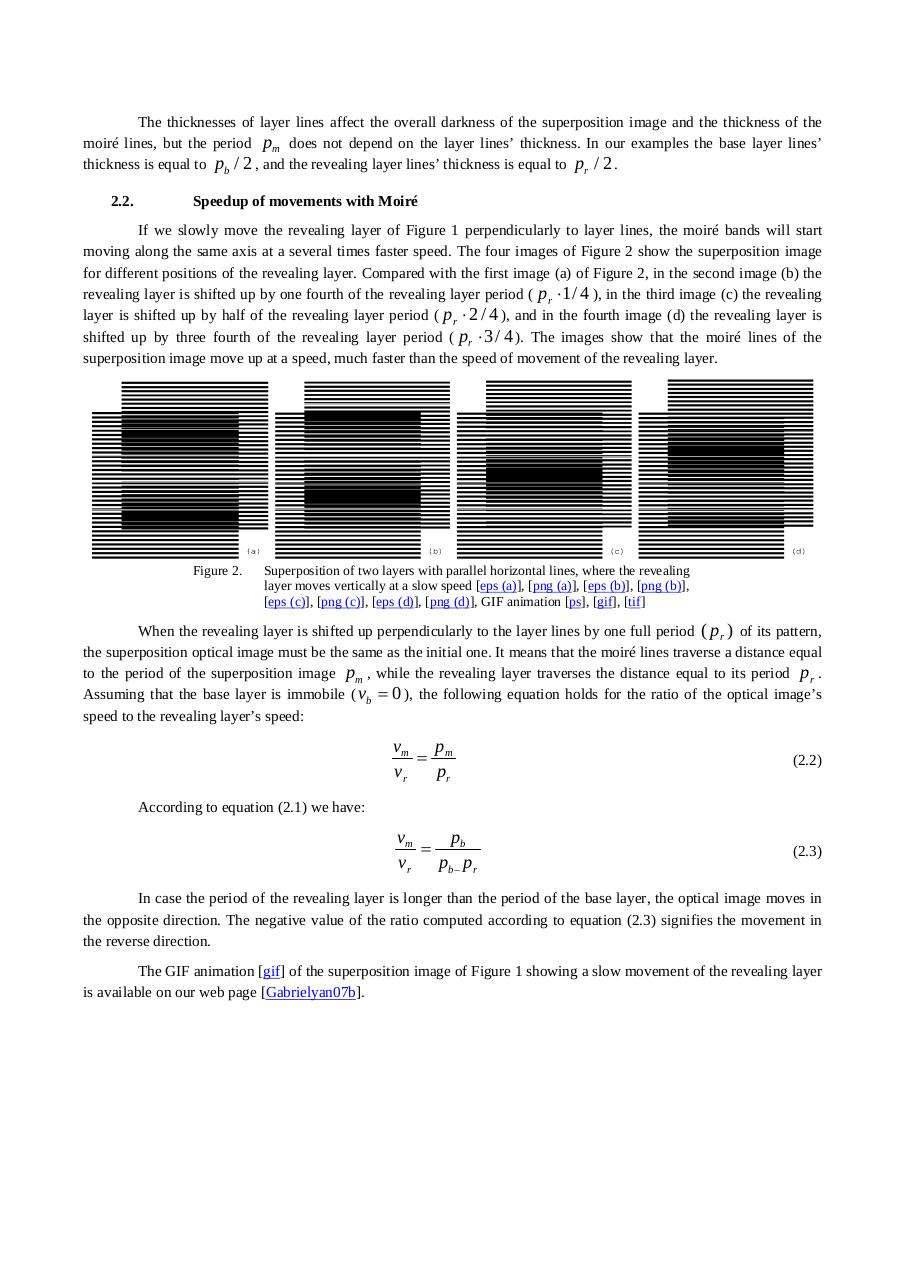# 10.1.1.399.1946.pdfPage 1 2 3 45624

#### Text preview

The thicknesses of layer lines affect the overall darkness of the superposition image and the thickness of the
moiré lines, but the period pm does not depend on the layer lines’ thickness. In our examples the base layer lines’
thickness is equal to pb / 2 , and the revealing layer lines’ thickness is equal to pr / 2 .
2.2.

Speedup of movements with Moiré

If we slowly move the revealing layer of Figure 1 perpendicularly to layer lines, the moiré bands will start
moving along the same axis at a several times faster speed. The four images of Figure 2 show the superposition image
for different positions of the revealing layer. Compared with the first image (a) of Figure 2, in the second image (b) the
revealing layer is shifted up by one fourth of the revealing layer period ( pr ⋅ 1 / 4 ), in the third image (c) the revealing
layer is shifted up by half of the revealing layer period ( pr ⋅ 2 / 4 ), and in the fourth image (d) the revealing layer is
shifted up by three fourth of the revealing layer period ( pr ⋅ 3 / 4 ). The images show that the moiré lines of the
superposition image move up at a speed, much faster than the speed of movement of the revealing layer.

Figure 2.

Superposition of two layers with parallel horizontal lines, where the revealing
layer moves vertically at a slow speed [eps (a)], [png (a)], [eps (b)], [png (b)],
[eps (c)], [png (c)], [eps (d)], [png (d)], GIF animation [ps], [gif], [tif]

When the revealing layer is shifted up perpendicularly to the layer lines by one full period ( pr ) of its pattern,
the superposition optical image must be the same as the initial one. It means that the moiré lines traverse a distance equal
to the period of the superposition image pm , while the revealing layer traverses the distance equal to its period pr .
Assuming that the base layer is immobile ( vb = 0 ), the following equation holds for the ratio of the optical image’s
speed to the revealing layer’s speed:

vm p m
=
vr
pr

(2.2)

vm
pb
=
vr
pb− pr

(2.3)

According to equation (2.1) we have:

In case the period of the revealing layer is longer than the period of the base layer, the optical image moves in
the opposite direction. The negative value of the ratio computed according to equation (2.3) signifies the movement in
the reverse direction.
The GIF animation [gif] of the superposition image of Figure 1 showing a slow movement of the revealing layer
is available on our web page [Gabrielyan07b].